# Maximum likelihood estimation

## Introduction

By now, you must be aware of linear regression. If not, you may follow the link before moving further. In linear regression, our primary objective was to decide the parameters in order to obtain the best fit line that could predict a data point with the least error. To obtain these parameters, we used Ordinary Least Squares(OLS) to check which set of parameters gave the best results.

Much like OLS, Maximum likelihood estimation is also a parameter estimation technique. The basis of the technique is that it decides the parameters, given the sample, to maximize the likelihood for the data to occur.

## Maximum Likelihood Estimation

Now that we have a basic idea of what MLE is based upon, we can now dive into the details of it.

### Likelihood function

Remember, the objective is to maximize the likelihood of observing data given specific probability distribution and its parameters.

P(X | theta)

Which could also be written as

Maximize{L(X | theta)}.

Here theta is an unknown parameter and is denoted with L.

So to attain the Maximum likelihood function we Maximize{L(X | theta)}.

The joint probability distribution is redefined as the product of the conditional probability for observing each example given the distribution parameters.

L(X | theta) = π(i to n) P (xi | theta)

### Log of likelihood

It’s going to be a lot of work taking the product of all these conditional probabilities. So, to make it slightly easier, we can take log(natural log) on both sides

ln L(X | theta) = ln(π(i to n) P (xi | theta))

Which becomes

ln L(X | theta) = ∑(i to n) log P(xi | theta)

MLE is an optimisation technique which can be used on various machine learning models like Logistic regression, linear regression, etc.

## MLE in statistical models

Conditional MLE for a supervised learning model can be given as:-

Maximise{∑(i to n) log P(xi ; h)}

Where h is the modelling hypothesis which replaces the model parameters. ‘h’ can be any supervised learning model we’re trying to optimise.

## Maximum Likelihood Estimation in Logistic Regression

The objective here would be to predict the best sigmoid curve for the given observation. And for that we need to find the best parameters. For that, we’ll use MLE.

Let the required cost function be given by P(Y;z). Where Y is our sample data and z is the unknown parameter.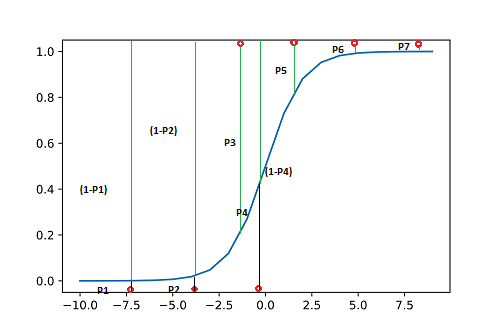Here, we have 7 points with respective probabilities their respective probabilities. For points to be 0 we need P1, P2, P4 to be as low as possible and for points to be 1, we need probabilities P3, P5, P6 and P7 to be as high as possible.

This may also be restated as if we need the product

(1-P1)*(1-P2)* P3*(1-P4)*P5*P6*P7

to be maximized. This is called the joint probability. The cost function may be written as-

J(z) = π(i to n) P (Yi ; z) (for n samples)

ln J(z) = ln(π(i to n) P (Yi ; z)) (Taking natural logs)

ln J(z) =L(z|Yi) = ∑(i to n) ln P (Yi ; z))

For a given value of z and the corresponding sample Yi, the function gives the probability of obtaining the observed values. If Yi=1 ,function becomes z. For Yi=0, the function becomes 1-z.

ln J(z) =L(z|Yi)=  ∑(i to n) ln (zyi *(1-z)1-yi ))

Simplifying it further, the final expression comes out to be-

The function maximizes at z= ∑(i=1 to n) Yi/n

## Implementation

``````# import the necessary libraries
import numpy as np
import pandas as pd
from matplotlib import pyplot as plt
import seaborn as sns
from statsmodels import api
from scipy import stats
from scipy.optimize import minimize``````

``````# create an independent variable
x = np.linspace(-10, 30, 100)
# create a normally distributed residual
e = np.random.normal(10, 5, 100)
# generate ground truth
y = 10 + 4*x + e
df = pd.DataFrame({'x':x, 'y':y})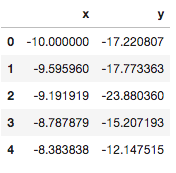``````# visualize data distribution
sns.regplot(x='x', y='y', data = df)
plt.show()``````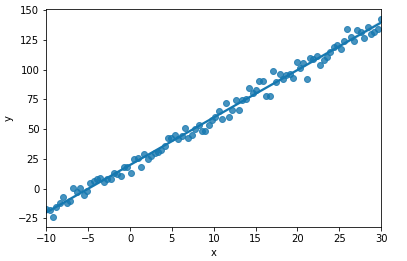BY OLS APPROACH

``````features = api.add_constant(df.x)
model = api.OLS(y, features).fit()
model.summary()``````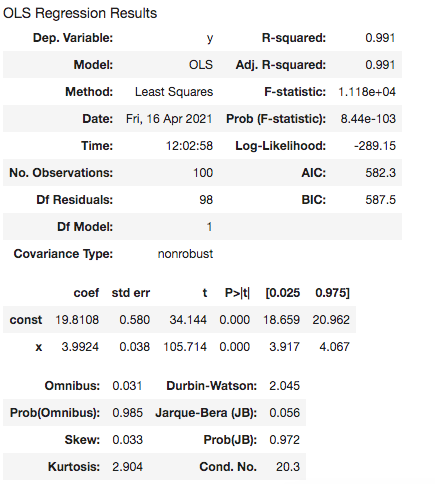``````# find the std dev
res = model.resid
standard_dev = np.std(res)
standard_dev``````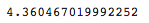BY MLE APPROACH

``````def MLE_Norm(parameters):
const, beta, std_dev = parameters
pred = const + beta*x

LL = np.sum(stats.norm.logpdf(y, pred, std_dev))
neg_LL = -1*LL
return neg_LL``````

``````mle_model = minimize(MLE_Norm, np.array([2,2,2]), method='L-BFGS-B')
mle_model``````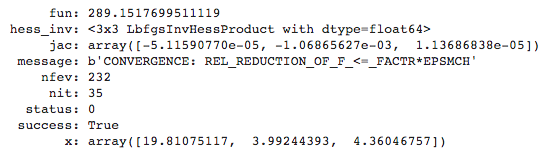The parameters obtained via both the approaches are similar.

## FAQs

1. Contrast Maximum likelihood estimation with ordinary least squares in linear regression.
The MLE chooses parameters that can maximize the likelihood or, equivalently the log-likelihood function. It then fits the model based on the trial estimated parameter value and calculate the mean of the model. To find the iterative weighted and working dependence and based on this two and the design matrix we can estimate the best parameter value.
OLS checks and minimizes the residual errors(square of the difference between observed value and the predicted value) of the model.

2. Provide an expression for maximum likelihood estimation in linear regression.
Without getting too much into the derivation, the final expression can be given as
Maximize {∑(i to n) log (1 / √(2 *π*sigma2)) – (1/(2 *sigma2) * (yi – h(xi, Beta))2)}
xi is a given example and beta is the coefficients of the linear regression model.

3. State the advantages of MLE over other estimators.
Following are the advantages of MLE over other estimators:
→ If model assumptions are right, it is the most efficient parameter estimation technique.
→ Provides a flexible approach suitable for a variety of applications.
→ Works the best for larger samples.

## Key Takeaways

Maximum likelihood estimation is a popular and widely used optimisation technique among data scientists. Maximum likelihood estimation chooses parameters in such a way that it maximizes the likelihood of observing the datapoints. Although, most companies might not expect a beginner to be aware of the nitty-gritty of this technique, an extra bit of knowledge always goes a long way. You may check out our industry-oriented machine learning courses curated by industry experts.